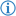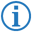The not operator is a Boolean operator which returns `true` if the argument is `false` and vice versa.

Its token is named NOT.

Remember that all comparison instructions do not need a not operator because there is always another operator which complements them:

A NOT A
Equal Not equal
Greater than Less than or equal
Less than Greater than or equal

1 Input
Input Output
T F
F T

## FDS### Example

`I did this as long as`` ``not`` ``Applejack`` ``is`` ``1``:` `...`

This loops the next part of code if variable `Applejack` is not equal to `1`.

This is equivalent to the following Java code:

```while(! (Applejack==1)) { ...
```

Remember that this code is equivalent to using not equal, such that:

`I did this as long as`` ``Applejack`` ``is not`` ``1``:`

and to the following Java code:

```while(Applejack!=1) { ...
```